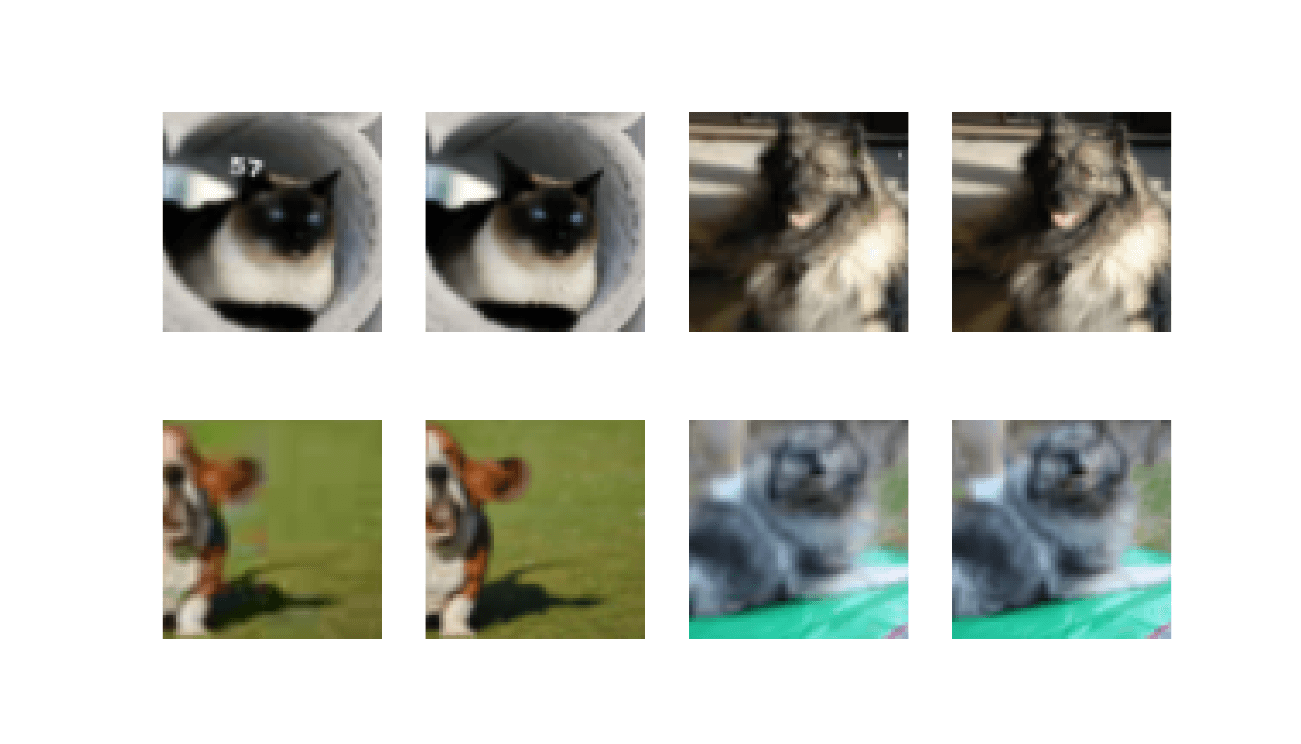## Intro

The fastai library simplifies training fast and accurate neural nets using modern best practices. See the fastai website to get started. The library is based on research into deep learning best practices undertaken at fast.ai, and includes “out of the box” support for vision, text, tabular, and collab (collaborative filtering) models.

## Dataset

Grab the pets dataset and Specify folders:

URLs_PETS()

path = 'oxford-iiit-pet'
path_hr = paste(path, 'images', sep = '/')
path_lr = paste(path, 'crappy', sep = '/')

Prepare the input data by crappifying images:

# run this only for the first time, then skip
items = get_image_files(path_hr)
parallel(crappifier(path_lr, path_hr), items)

bs = 10
size = 64
arch = resnet34()

get_dls = function(bs, size) {
dblock = DataBlock(blocks = list(ImageBlock, ImageBlock),
get_items = get_image_files,
get_y = function(x) {paste(path_hr, as.character(x$name), sep = '/')}, splitter = RandomSplitter(), item_tfms = Resize(size), batch_tfms = list( aug_transforms(max_zoom = 2.), Normalize_from_stats( imagenet_stats() ) )) dls = dblock %>% dataloaders(path_lr, bs = bs, path = path) dls$c = 3L
dls
}

dls_gen = get_dls(bs, size)

See batch:

dls_gen %>% show_batch(max_n = 4, dpi = 150)## Fit

Define loss function and create unet_learner:

wd = 1e-3

y_range = c(-3.,3.)

loss_gen = MSELossFlat()

create_gen_learner = function() {
unet_learner(dls_gen, arch, loss_func = loss_gen,
config = unet_config(blur=TRUE, norm_type = "Weight",
self_attention = TRUE, y_range = y_range))
}

learn_gen = create_gen_learner()

learn_gen %>% fit_one_cycle(2, pct_start = 0.8, wd = wd)
epoch     train_loss  valid_loss  time
0         0.025911    0.035153    00:42
1         0.019524    0.019408    00:39

## Conclusion

Plot results:

learn_gen %>% show_results(max_n = 6, dpi = 200)